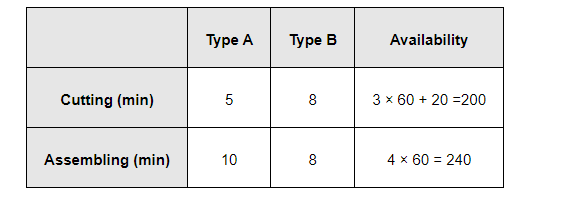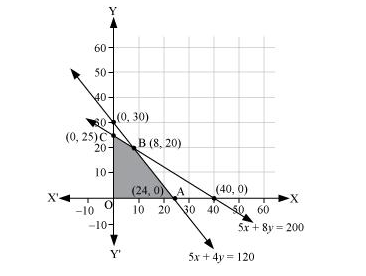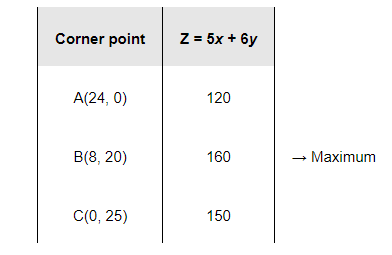# A company manufactures two types of novelty souvenirs made of plywood.

Question:

A company manufactures two types of novelty souvenirs made of plywood. Souvenirs of type A require 5 minutes each for cutting and 10 minutes each for assembling. Souvenirs of type B require 8 minutes each for cutting and 8 minutes each for assembling. There are 3 hours 20 minutes available for cutting and 4 hours of assembling. The profit is Rs 5 each for type A and Rs 6 each for type B souvenirs. How many souvenirs of each type should the company manufacture in order to maximize the profit?

Solution:

Let the company manufacture x souvenirs of type A and y souvenirs of type B. Therefore,

x ≥ 0 and y ≥ 0

The given information can be complied in a table as follows.The profit on type A souvenirs is Rs 5 and on type B souvenirs is Rs 6. Therefore, the constraints are

$5 x+8 y \leq 200$

$10 x+8 y \leq 240$ i.e., $5 x+4 y \leq 120$

The mathematical formulation of the given problem is

Maximize $Z=5 x+6 y$       ...(1)

subject to the constraints,

$5 x+8 y \leq 200 \ldots(2)$

$5 x+4 y \leq 120 \ldots(3)$

$x, y \geq 0 \ldots(4)$

The feasible region determined by the system of constraints is as follows.The corner points are A (24, 0), B (8, 20), and C (0, 25).

The values of Z at these corner points are as follows.The maximum value of Z is 200 at (8, 20).

Thus, 8 souvenirs of type A and 20 souvenirs of type B should be produced each day to get the maximum profit of Rs 160.